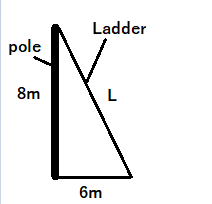mycollegehive
The foot of a ladder is 6m from the base of an electric pole...
waec-2019 ssce-exam-2019 waec-mathematics-2019 math0The foot of a ladder is 6m from the base of an electric pole. The top of the ladder rest against the pole at a point 8m above the ground. How long is the ladder

A. 14m

B. 12m

C. 10m

D. 7m

776 viewsShareFollowUniversity of Lagos Nigeria
22 April 2020University of Benin Nigeria
20 July 20200The diagram above is a right angle triangle, therefore pythagoras theorem can be used to find the length, L of the ladder

From pythagoras theorem, hyp2 = opp2 + adj2

hyp, stands for hypotenus = L

opp, stands for opposite = 8

Length of the ladder, L = √82 + 62

= √100

= 10mShare

### Groups

How to insert math formulas/equations### Related Tags

waec-2019

14 followers

64 questionsssce-exam-2019

10 followers

64 questionswaec-mathematics-2019

15 followers

53 questionsmath

44 followers

1303 questions